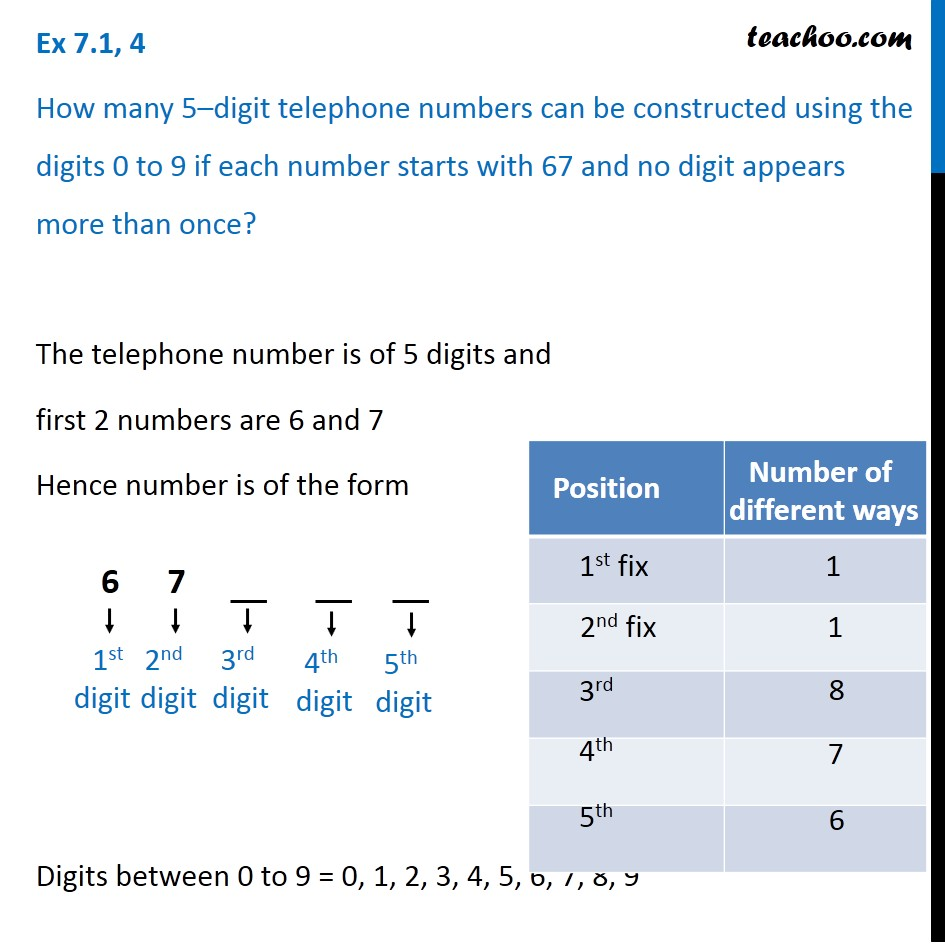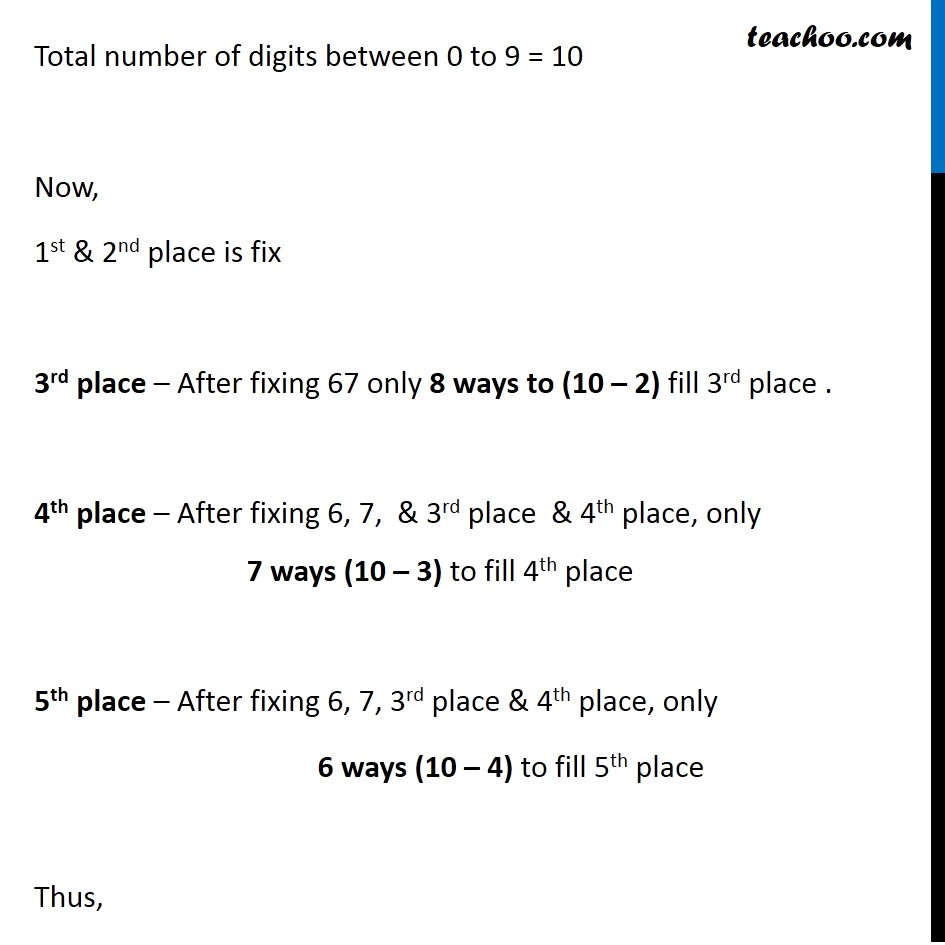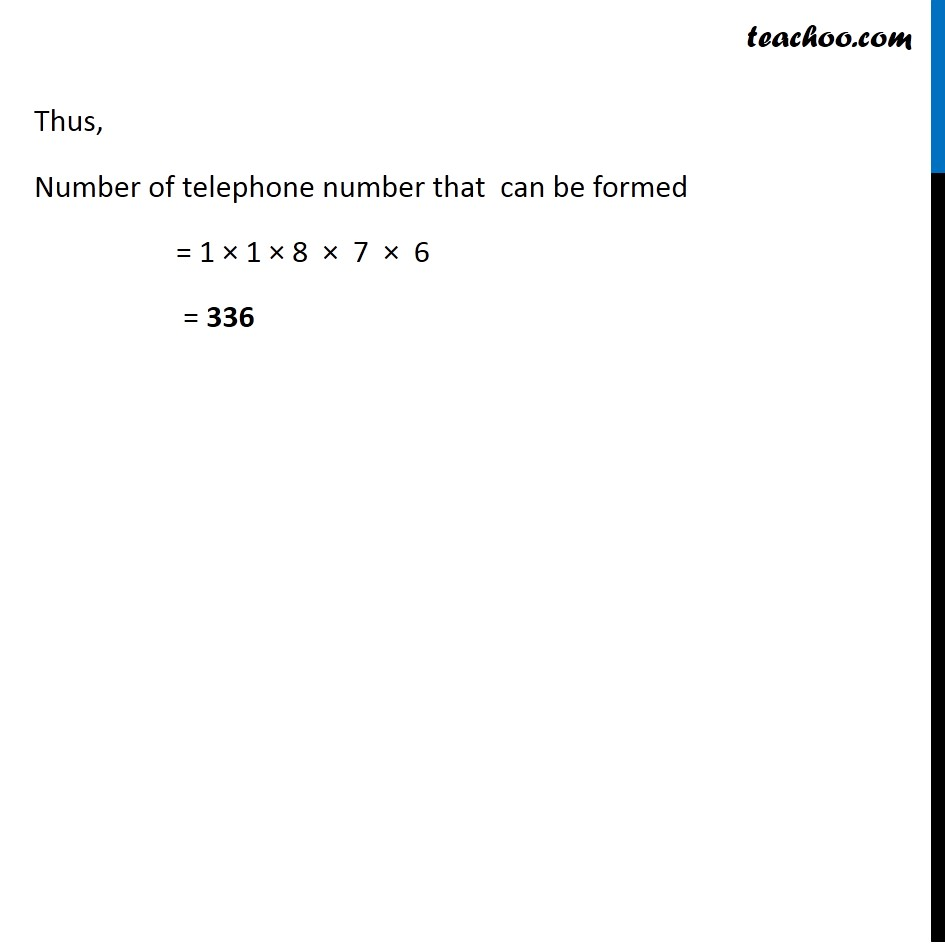1. Chapter 7 Class 11 Permutations and Combinations (Term 2)
2. Serial order wise
3. Ex 7.1

Transcript

Ex 7.1, 4 How many 5–digit telephone numbers can be constructed using the digits 0 to 9 if each number starts with 67 and no digit appears more than once? The telephone number is of 5 digits and first 2 numbers are 6 and 7 Hence number is of the form Digits between 0 to 9 = 0, 1, 2, 3, 4, 5, 6, 7, 8, 9 1st digit 2nd digit 3rd digit 4th digit 5th digit Total number of digits between 0 to 9 = 10 Now, 1st & 2nd place is fix 3rd place – After fixing 67 only 8 ways to (10 – 2) fill 3rd place . 4th place – After fixing 6, 7, & 3rd place & 4th place, only 7 ways (10 – 3) to fill 4th place 5th place – After fixing 6, 7, 3rd place & 4th place, only 6 ways (10 – 4) to fill 5th place Thus, Number of telephone number that can be formed = 1 × 1 × 8 × 7 × 6 = 336 Thus, Number of telephone number that can be formed = 1 × 1 × 8 × 7 × 6 = 336

Ex 7.1

Chapter 7 Class 11 Permutations and Combinations (Term 2)
Serial order wise